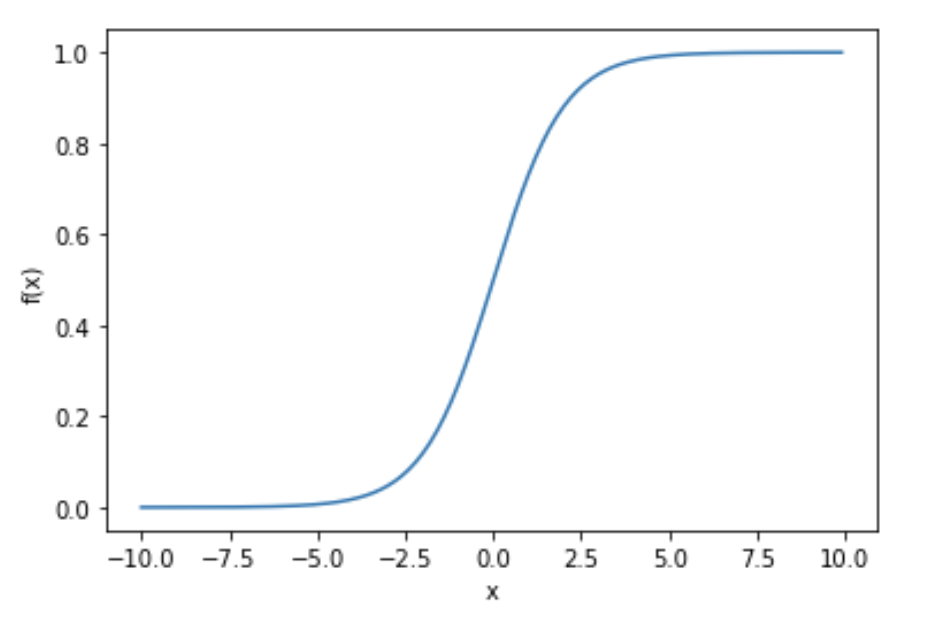•Krishna Kankipati

# Artificial Neural Networks (Part 1)-Role of Activation Functions, Weights and Bias.

Artificial Neural Network(ANN) is a software implementation of neural structure of Human brain. The human brain is a complex network of Neurons connected to each other, which process output when simulated.

## Artificial Neuron

The neuron consists of a summation function along with an activation function.An Artificial Neuron

The neuron is stimulated by an Activation Function such as Sigmoid, Tanh, ReLU, LeakyReLu…

Implementing Sigmoid function in PythonCodeSigmoid Function Graph

We can see that the Sigmoid function (f(x)) isn’t a step function, the edge is soft, i.e a derivative can be applied on this function. The derivative of the function helps at the time of model training.

In general a neuron takes an input and outputs some value. The node takes multiple weighted inputs, summate the inputs and then applies an activation function i.e turning a Linear function into a nonlinear function and thus generates an output.Node

Here each input is associated with weights. As we’ve seen that the Artificial Neuron consists of two segments a summation function(y) and an activation function(f).

y = X1W1 + X2W2 + X3W3 + b

w1, w2, w3 are the weights(real numbers) which are changed during the learning process of an algorithm. These help in determining the input and the slope of the graph. ‘b’ is a bias term, it helps in determining the output of the node.

The above equation is similar to a function we have learned in our school days :

y = mx+c.

The activation function is represented as following

f(y)

## Let us look how weights and bias affect the activation function(sigmoid function)

When we change the weights associated with the inputs, the slope of the function changes, i.e the selection part of the data changes when an algorithm is in training/learning process.CodeSigmoid function with varying weights

This change in slope helps in better understanding of the relation between the input and output variables.

Okay, what if we want to change the output based on a condition like when x > 0 or x <0?CodeVariation in Sigmoid function with varying bias values

The bias term helps to apply conditional relations such that a node can fire the output based on that condition.

Thus we have learned how weights and bias help a model to learn the hidden relations between the feature values and target values. In Part 2, we will take a look at a simple neural network structure and implement it in python.

See All